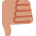# Costing & Revenue -Budgeted Breakeven

Hi,

Can someone please explain the following to me as I am totally lost and I have an exam coming upThe following is an answer from an online AAT exam I done the exam and got this question wrong so I downloaded the answer to have a look at it and I cannot for the life of me figure out how they have came to this answer:

Direct materials £6.25
Direct Labour £4.10
Total Variable Costs £14.00
Total Costs £26.00

Product LN60 has a selling price of £39 per unit. Budgeted sales volume is 5000 units.

Question: (b) Calculate the breakeven volume in units for LN60

Answer: The breakeven volume for LN60 is 2400 units

SherrieMarie

• Hi SherrieMarie,

I cannot for the life of me figure out how they got the answer either, based on the limited information that we've been given here.

The breakeven volume is the sales volume that must be attained in order for the total costs to equal the total revenues. So basically, the volume at which we're not making a loss or a profit. We can calculate our revenues easily enough, the problem is, how do we work out the sales revenues or sales volume that we need, if we don't actually know what our costs are? We haven't been given the figure for our total fixed costs.

The variable costs will go up proportionally with each unit that we produce e.g. we produce and sell say 5,000 units (revenues of £195,000) then our variable costs are 5,000 units x £14 variable cost per unit ( which equals £70,000). What are the fixed costs for 5,000 units? Based on the information above, we don't know, so it's the total fixed costs figure that we need in order to work out the breakeven volume.

If we had been given our total fixed costs then the calculation would go like this:

Total fixed costs
---------------- = Breakeven volume
Contribution per unit

How many sales units will be required to break even? Since costs go up proportionally, with each additional unit of LN60 produced anyway (like the revenues - we assume that bulk discounts don't exist-), the variable costs aren't relevant here to working out the units required to break even. We will take our variable costs out of the equation by working out the contribution:
Contribution = unit selling price - unit variable cost

Many people confuse contribution with profit. Our contribution is basically 'paying off' our fixed costs. Once this is done, then we start making profits.

The contribution in this case is:
£39 - £14 = £25

Apply the contribution to the above equation.
_____________________________________________________________________________________

The answer you gave me for the breakeven volume was 2,400 units. So, the missing piece of information that you should have been given I will work out as follows;

2,400 units x £12 (fixed costs per unit) = £28, 800 Should be our total fixed costs and it's the figure that you should have been given.

I hope this helps and don't hesitate to ask any questions.
• But you are given the fixed cost, 5,000 units @ £12 = £60,000
Contribution per unit = £39-£14 = £25
break even volume = £60,000/£25 = 2,400 units
• Thank you for your help• The trick in this section of CSTR is very often the budgeted sales volume/sales forecast.

The information is usually set apart from the other numerical information, perhaps above, perhaps below, hidden in the verbiage.

As a result, exam candidates often fail to spot it.

In this question, as in stevef's correct calculation, once you spot it, the rest is straightforward.
• i know you have seen the answer and may have figure out how to solve this.
i will still show you my way of doing. as we know BEP= total fixed cost/contribution. so in this case we know the unit cost of all and the total units being 5000 units.
so, total fixed= 12*5000=60,000 ( add if the question ask you for extra profit plan)
contribution per unit= sp-vc = 39-14=25
so BEP=60,000/25=2,400 units
Good luck